# 31 Year NEET Previous Year Questions: Motion in a Straight Line - 1

## 16 Questions MCQ Test Physics 31 Years NEET Chapterwise Solved Papers | 31 Year NEET Previous Year Questions: Motion in a Straight Line - 1

Description
Attempt 31 Year NEET Previous Year Questions: Motion in a Straight Line - 1 | 16 questions in 30 minutes | Mock test for NEET preparation | Free important questions MCQ to study Physics 31 Years NEET Chapterwise Solved Papers for NEET Exam | Download free PDF with solutions
QUESTION: 1

### A particle moving along x-axis has acceleration f, at time t, given by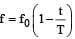, where f0 and T are constants. The particle at t = 0 has zero velocity. In the time interval between t = 0 and the instant when f = 0, the particle’s velocity (vx) is 

Solution:

Here,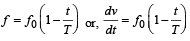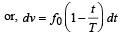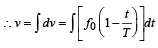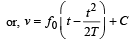where C is the constant of integration.
At t = 0, v = 0.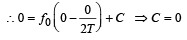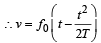If f = 0, then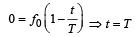Hence, particle's velocity in the time interval t = 0 and t = T is given by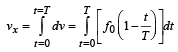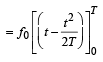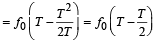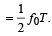QUESTION: 2

### The distance travelled by a particle starting from rest and moving with an acceleration 4/3ms-2 , in the third second is:

Solution:

Distance travelled in the nth second is given by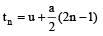put u = 0,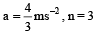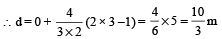QUESTION: 3

###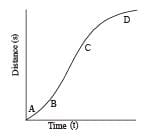A particle shows distance - time curve as given in this figure. The maximum instantaneous velocity of the particle is around the point:                              

Solution:

The slope of the graph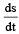is maximum at C and hence the instantaneous velocity is maximum at C.

QUESTION: 4

A particle moves in a straight line with a constant acceleration. It changes its velocity from 10 ms–1 to 20 ms–1 while passing through a distance 135 m in t second. The value of t is: 

Solution:

Initial velocity, u = 10 ms–1
Final velocity, v = 20 ms–1
Distance, s = 135 m
Let, acceleration = a
Using the formula, v2 = u2 + 2as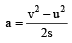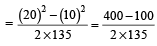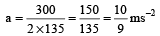Now, using the relation, v = u + at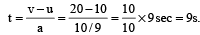QUESTION: 5

A bus is moving with a speed of 10 ms–1 on a straight road. A scooterist wishes to overtake the bus in 100 s. If the bus is at a distance of 1 km from the scooterist, with what speed should the scooterist ch ase the bus? 

Solution:

Let v be the relative velocity of scooter w.r.t bus as v = vS– vB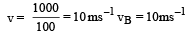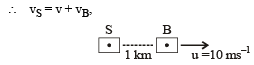=   10 + 10 = 20 ms–1

∴ velocity of scooter = 20 ms–1

QUESTION: 6

A particle starts its motion from rest under the action of a constant force. If the distance covered in first 10 seconds is S1 and that covered in the first 20 seconds is S2, then: 

Solution:

u = 0, t1=10s, t2 = 20s

Using the relation,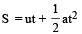Acceleration being the same in two cases,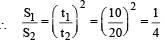S2 = 4S1

QUESTION: 7

A ball is dropped from a high rise platform at t = 0 starting from rest. After 6 seconds another ball is thrown downwards from the same platform with a speed v. The two balls meet at t = 18s.What is the value of v?  (take g = 10 m/s2)

Solution:

Clearly distance moved by 1st bal l in 18s = distance moved by 2nd ball in 12s.
Now, distance moved in 18 s by 1st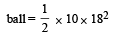= 90 x 18 = 1620 m

Distance moved in 12s by 2nd ball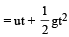∴ 1620 = 12 v + 5 x 144

⇒    v = 135 – 60 = 75 ms –1

QUESTION: 8

A particle h as in itial velocity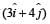and has acceleration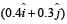. It's speed after 10 s is:

Solution: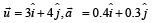⇒ ux = 3 units, uy = 4 units

ax = 0.4 units, ay = 0.3 units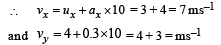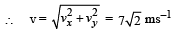QUESTION: 9

A particle moves a distance x in time t according to equation x = (t + 5)-1. The acceleration of particle is proportional to 

Solution: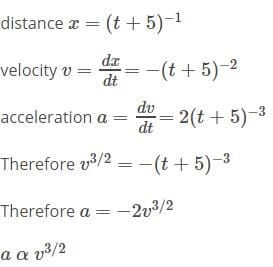QUESTION: 10

A man of 50 kg mass is standing in a gravity free space at a height of 10 m  above the floor. He throws a stone of 0.5 kg  mass downwards with a speed 2 m/s. When the stone reaches the floor, the distance of the man above the floor will be:

Solution:

No external force is acting, there fore, momentum is conserved.
By momentum conservation, 50u + 0.5 × 2 = 0 where u is the velocity of man.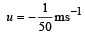Negative sign of u shows that man moves upward.
Time taken by the stone to reach the ground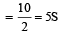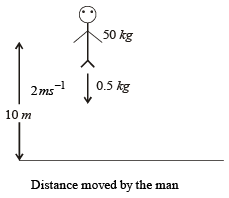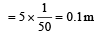∴ when the stone reaches the floor, the distance of the man above floor = 10.1 m

QUESTION: 11

A boy standing at the top of a tower of 20m height drops a stone. Assuming g = 10 ms–2, the velocity with which it hits the ground is 

Solution:

Here, u = 0

We have, v2 = u2 + 2gh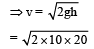= 20 m/s

QUESTION: 12

A body is moving with velocity 30 m/s towards east. After 10 seconds its velocity becomes 40 m/s towards north. The average acceleration of the body is 

Solution:

Average acceleration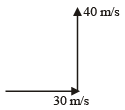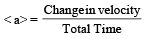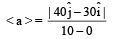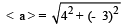< a > = 5 m/sec2

QUESTION: 13

A particle covers half of its total distance with speed v1 and the rest half distance with speed v2. Its average speed during the complete journey is

Solution:

Let the total distance covered by the particle be 2s. Then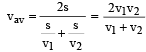QUESTION: 14

The motion of a particle along a straight line is described by equation :  x = 8 + 12t – t3 where x is in metre and t in second. The retardation of the particle when its velocity becomes zero, is :

Solution:

x = 8 + 12t – t3
The final velocity of the particle will be zero, because it retarded.
V = 0 + 12 – 3t2 = 0
3t2 = 12
t = 2 sec
Now the retardation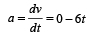a [t = 2] = – 12 m/s2 retardation = 12 m/s2

QUESTION: 15

A stone falls freely  under gravity. It covers distances h1, h2 and h3 in the first 5 seconds, the next 5 seconds and the next 5 seconds respectively. The relation between h1, h2 and h3 is [NEET 2013]

Solution: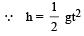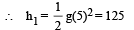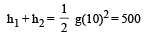⇒  h2 = 375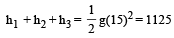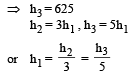QUESTION: 16

The displacement ‘x’ (in meter) of a particle of mass ‘m’ (in kg) moving in one dimension under the action of a force, is related to time ‘t’ (in sec) by t =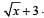. The displacement of the particle when its velocity is zero, will be [NEET Kar. 2013]

Solution: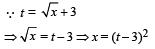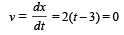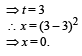Use Code STAYHOME200 and get INR 200 additional OFF Use Coupon Code